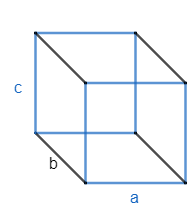QUESTION

# Area of the four walls of a room is $108{{m}^{2}}$ . If the height and length of the room are in the ratio of 2:5 and the height and breadth in the ratio 4:5, then the area in ${{m}^{2}}$ of the floor of the room is?a) 72b) 54c) 45d) 24

Hint: Area of four walls (lateral surface area) of a cuboid is given by the relation; 2c (a + b) where a, b, c are the length, breadth and height of the cuboid respectively. Find the equations with the help of given ratios of height, breadth and height, length. Solve them to get the dimensions of the cuboid and hence find the area of base. Use the area of the rectangle for calculating the area of base as $\text{Area of rectangle}=\text{length}\times \text{breadth}$.

As we know room is of cuboid shape in the given problem and we have the area of four walls of the room as $108{{m}^{2}}$ and ratio of height, length is 2:5 and ratio of height, breadth is 4:5, so we need to determine the area of floor.
Diagram of the floor can be given asNow, we can observe that walls will contain the side four faces of the room. Hence area of the walls can be given by using the area of rectangle which can be given as
$\text{Area of rectangle}=\text{length}\times \text{breadth}..........\left( i \right)$
Hence, area of four walls of the room is given as
Area of walls $=c\times b+c\times a+c\times b+c\times a=2c\left( a+b \right).............\left( ii \right)$
Where a, b, c are the length, breadth and height of the given room. Now, the area of walls is given as $108{{m}^{2}}$ in the problem. So, we get equation as
2c (a + b) = 108 or c (a + b) = 54 ……………….(iii)
Now, it is given that the ratio of height and length is 2:5 and ratio of height and breadth is 4:5. Hence, we can get equation in terms of a, b, c as
$\dfrac{c}{a}=\dfrac{2}{5},\dfrac{c}{b}=\dfrac{4}{5}$
Now, we can get values of a and b in terms of c from both the equations by cross multiplying the terms: so, we get
5c = 2a and 5c = 4b or
$a=\dfrac{5c}{2},b=\dfrac{5c}{4}................\left( iv \right)$
Now, put the values of ‘a’ and ‘b’ in the equation (iii) from the equation (iv). So, we get
\begin{align} & c\left( \dfrac{5c}{2}+\dfrac{5c}{4} \right)=54 \\ & c\left( \dfrac{10c+5c}{4} \right)=54 \\ & c\left( \dfrac{15c}{4} \right)=54 \\ & 15{{c}^{2}}=4\times 54 \\ \end{align}
Hence, we get
\begin{align} & {{c}^{2}}=\dfrac{4\times 54}{15}=\dfrac{4\times 18}{5}=\dfrac{72}{5} \\ & c=\sqrt{\dfrac{72}{5}}=\sqrt{\dfrac{36\times 2}{5}}=6\sqrt{\dfrac{2}{5}} \\ \end{align}
Now, we can get values of a and b from the equation (iv) as
\begin{align} & a=\dfrac{5}{2}\times 6\sqrt{\dfrac{2}{5}}=15\sqrt{\dfrac{2}{5}} \\ & b=\dfrac{5}{4}\times 6\sqrt{\dfrac{2}{5}}=\dfrac{15}{2}\sqrt{\dfrac{2}{5}} \\ \end{align}
Now, we can get the area of the floor by using the equation (i) as the floor is of shape of rectangle as well. So, we get
Area of floor $=a\times b=15\sqrt{\dfrac{2}{5}}\times \dfrac{15}{2}\sqrt{\dfrac{2}{5}}=\dfrac{225}{2}\times \dfrac{2}{5}=45{{m}^{2}}$
Hence, option (c) is the correct answer.

Note: One may go wrong if he or she uses the area of cuboid as 2 (lb + bh + hl) where l, b, h are the length, breadth, height respectively. As it is representing the total area of the cuboid not the area of four walls. We need to remove the area of upper and lower face from the above mentioned formula i.e. 2lb. So, we get an area of walls (termed as lateral surface area) as 2h(l + b).
Another approach for solving the problem would be that we can assume the height and length of the room as 2x and 5x as the ratio between them is 2:5. Similarly, height and breadth can be taken as 4y and 5y respectively as the ratio between them is 4:5. Now, equate heights and get relation of x and y, now put the dimensions in the formula of area of walls in terms of ‘x’ or ‘y’ (only one variable) to get the answer. So, it can be another approach as well. Don’t solve $\sqrt{\dfrac{2}{5}}$ in decimal form for the length and breadth as the root will disappear once they get multiplied.